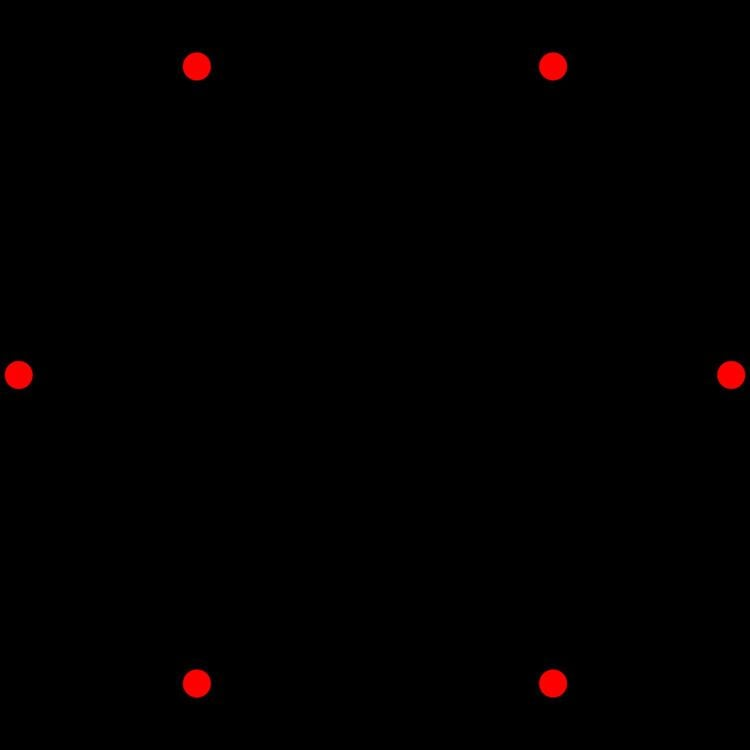# A5 polytope

Updated on
Covid-19In 5-dimensional geometry, there are 19 uniform polytopes with A5 symmetry. There is one self-dual regular form, the 5-simplex with 6 vertices.

Each can be visualized as symmetric orthographic projections in Coxeter planes of the A5 Coxeter group, and other subgroups.

## Graphs

Symmetric orthographic projections of these 19 polytopes can be made in the A5, A4, A3, A2 Coxeter planes. Ak graphs have [k+1] symmetry. For even k and symmetrically nodea_1ed-diagrams, symmetry doubles to [2(k+1)].

These 19 polytopes are each shown in these 4 symmetry planes, with vertices and edges drawn, and vertices colored by the number of overlapping vertices in each projective position.

## References

Similar Topics
The Woman He Married
Herman Makarenko
Amanda Havard
Topics